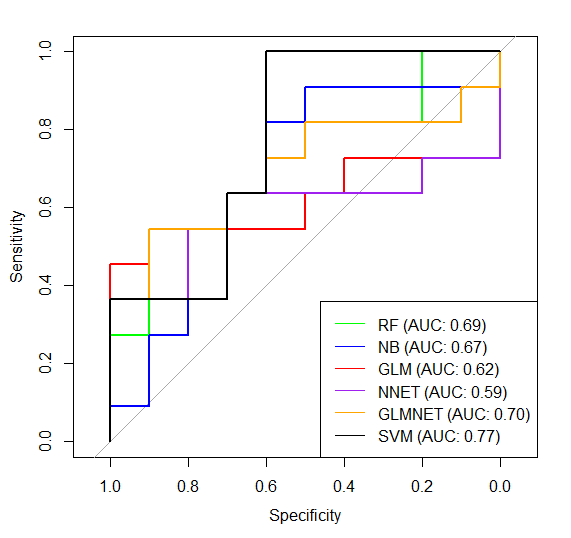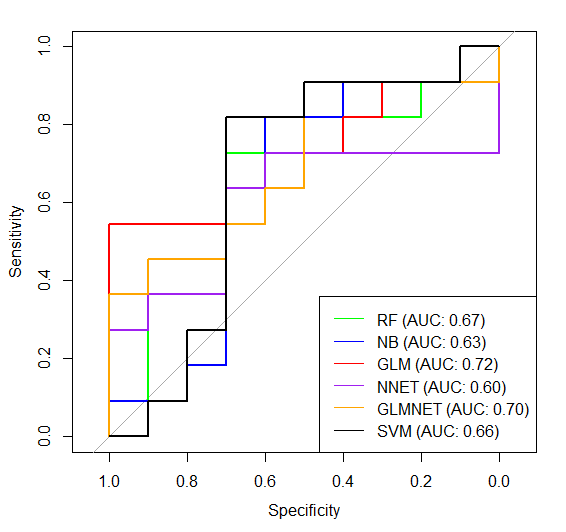# #StackBounty: #r #machine-learning #caret Best model (set of predictors) for my data?

### Bounty: 50

I’m exploring some ML strategies using `caret` package. My goal is to select best predictors and to obtain optimal model for further predictions. My dataset is:

• 75 observations (39 S and 36 F – dependent variable named ‘group’) – dataset is well balanced
• 13 independent variables (predictors), continous values from 0 to 1, without any NA’s named:

A_1, A_2, A_3, A_4, A_5, B_1, B_2, C_1, C_2, C_3, D_1, D_2, E_1

Moreover, values of each predictor (F vs S) significantly differ (Wilcoxon test).

I started division of the data and 10-fold cross validation:

``````set.seed(355)
trainIndex <- createDataPartition(data\$group, p = 0.7, list = FALSE)
trainingSet <- data[trainIndex,]
testSet <- data[-trainIndex,]

methodCtrl <- trainControl(
method = "repeatedcv",
number = 10,
repeats = 5,
savePredictions = "final",
classProbs = T,
summaryFunction = twoClassSummary
)
``````

Then, based on several articles and tutorials I selected six ML methods to obtain some models with all predictor variables:

``````rff <- train(group ~., data = trainingSet, method = "rf", metric = "ROC", trControl = methodCtrl)
nbb <- train(group ~., data = trainingSet, method = "nb", metric = "ROC", trControl = methodCtrl)
glmm <- train(group ~., data = trainingSet, method = "glm", metric = "ROC", trControl = methodCtrl)
nnett <- train(group ~., data = trainingSet, method = "nnet", metric = "ROC", trControl = methodCtrl)
glmnett <- train(group ~., data = trainingSet, method = "glmnet", metric = "ROC", trControl = methodCtrl)
svmRadiall <- train(group ~., data = trainingSet, method = "svmRadial", metric = "ROC", trControl = methodCtrl)
``````

How accurate are the models?

``````fitted <- predict(rff, testSet)
confusionMatrix(reference = factor(testSet$$group), data = fitted, mode = "everything", positive = "F")#61 #61 fitted <- predict(nbb, testSet) confusionMatrix(reference = factor(testSet$$group), data = fitted, mode = "everything", positive = "F")#66 #66
fitted <- predict(glmm, testSet)
confusionMatrix(reference = factor(testSet$$group), data = fitted, mode = "everything", positive = "F")#57 #66 fitted <- predict(nnett, testSet) confusionMatrix(reference = factor(testSet$$group), data = fitted, mode = "everything", positive = "F")#42 #66
fitted <- predict(glmnett, testSet)
confusionMatrix(reference = factor(testSet$$group), data = fitted, mode = "everything", positive = "F")#61 #57 fitted <- predict(svmRadiall, testSet) confusionMatrix(reference = factor(testSet$$group), data = fitted, mode = "everything", positive = "F")#66 #66
``````

After first `#` I put the % of accuracy of prediction of each model. I also draw simple ROC comparison of all models:Now I’d like to improve my model, so I used `glmStepAIC` to get only best (most important) predictors, here’s what I got:

``````aic <- train(group ~., data = trainingSet,  method = "glmStepAIC", trControl = methodCtrl, metric = "ROC", trace = FALSE)
summary(aic\$finalModel)

Deviance Residuals:
Min       1Q   Median       3Q      Max
-2.0191  -0.6077   0.3584   0.6991   2.5416

Coefficients:
Estimate Std. Error z value Pr(>|z|)
(Intercept)  0.39809    1.01733   0.391  0.69557
A_5          0.11726    0.04701   2.494  0.01263 *
C_2          0.17789    0.11084   1.605  0.10852
C_3         -0.18231    0.11027  -1.653  0.09828 .
E_1         -0.14176    0.05260  -2.695  0.00704 **
---
Signif. codes:  0 ‘***’ 0.001 ‘**’ 0.01 ‘*’ 0.05 ‘.’ 0.1 ‘ ’ 1

(Dispersion parameter for binomial family taken to be 1)

Null deviance: 74.786  on 53  degrees of freedom
Residual deviance: 48.326  on 49  degrees of freedom
AIC: 58.326

Number of Fisher Scoring iterations: 5
``````

Based on this result I chose this 4 predictor variables:

``````data <- read.table("data.txt", sep ='t',header = T, dec = ',')
data <- data[,c('group','A_5','C_2','C_3','E_1')]
``````

And I repeated everything, data division train – test, model obtain, model testing etc only with these 4 predictors instead of all 13. Unfortunately the accuracy is still low, take a look of % after second `#` in `confusionMatrix` part. Moreover the ROC comparison is even worse:I’m a new in such analysis, so could you please tell me if I’m making some bad mistake in my analysis or maybe my dataset is to small/my data is rubish?
How can I choose optimal predictors to get best model? Which ML methos should I pick?

Best Regards,